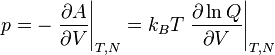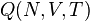# Difference between revisions of "Pressure"

Pressure ($p$) is the force per unit area applied on a surface, in a direction perpendicular to that surface, i.e. the scalar part of the stress tensor. The SI units for pressure are Pascals (Pa). In thermodynamics the pressure is given by$p = - \left.\frac{\partial A}{\partial V} \right\vert_{T,N} = k_BT \left.\frac{\partial \ln Q}{\partial V} \right\vert_{T,N}$
where$A$ is the Helmholtz energy function,$V$ is the volume,$k_B$ is the Boltzmann constant,$T$ is the temperature and$Q (N,V,T)$ is the canonical ensemble partition function.